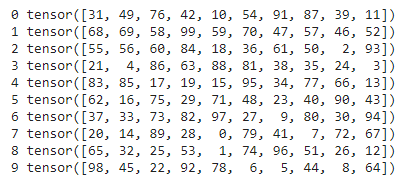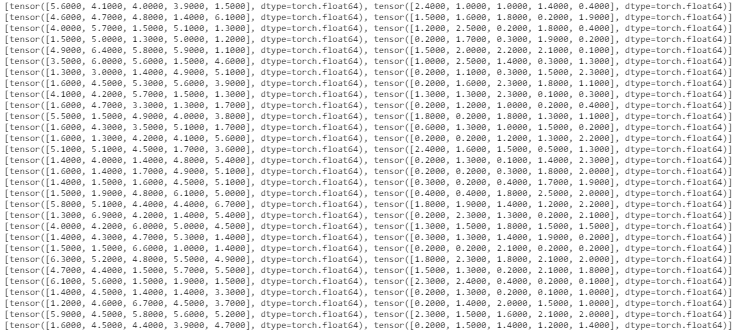# How to use a DataLoader in PyTorch?

• Last Updated : 24 Feb, 2021

Operating with large datasets requires loading them into memory all at once. In most cases, we face a memory outage due to the limited amount of memory available in the system. Also, the programs tend to run slowly due to heavy datasets loaded once. PyTorch offers a solution for parallelizing the data loading process with automatic batching by using DataLoader. Dataloader has been used to parallelize the data loading as this boosts up the speed and saves memory.

The dataloader constructor resides in the torch.utils.data package. It has various parameters among which the only mandatory argument to be passed is the dataset that has to be loaded, and the rest all are optional arguments.

Syntax:

To implement dataloaders on a custom dataset we need to override the following two subclass functions:

• The _len_() function: returns the size of the dataset.
• The _getitem_() function: returns a sample of the given index from the dataset.

## Python3

 `# importing the required libraries``import` `torch``from` `torch.utils.data ``import` `Dataset``from` `torch.utils.data ``import` `DataLoader`` ` `# defining the Dataset class``class` `data_set(Dataset):``    ``def` `__init__(``self``):``        ``numbers ``=` `list``(``range``(``0``, ``100``, ``1``))``        ``self``.data ``=` `numbers`` ` `    ``def` `__len__(``self``):``        ``return` `len``(``self``.data)`` ` `    ``def` `__getitem__(``self``, index):``        ``return` `self``.data[index]`` ` ` ` `dataset ``=` `data_set()`` ` `# implementing dataloader on the dataset and printing per batch``dataloader ``=` `DataLoader(dataset, batch_size``=``10``, shuffle``=``True``)``for` `i, batch ``in` `enumerate``(dataloader):``    ``print``(i, batch)`

Output:`# importing the required libraries``import` `torch``from` `torch.utils.data ``import` `Dataset``from` `torch.utils.data ``import` `DataLoader``import` `seaborn as sns``from` `torch.utils.data ``import` `TensorDataset`` ` `# defining the dataset consisting of ``# two columns from iris dataset``iris ``=` `sns.load_dataset(``'iris'``)``petal_length ``=` `torch.tensor(iris[``'petal_length'``])``petal_width ``=` `torch.tensor(iris[``'petal_width'``])``dataset ``=` `TensorDataset(petal_length, petal_width)`` ` `# implementing dataloader on the dataset ``# and printing per batch``dataloader ``=` `DataLoader(dataset, ``                        ``batch_size``=``5``, ``                        ``shuffle``=``True``)`` ` `for` `i ``in` `dataloader:``    ``print``(i)`